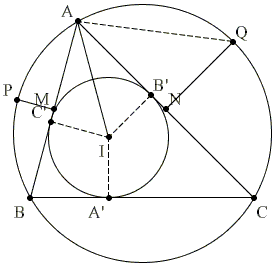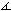# Sangaku with Versines

The following Sangaku has been written in 1825 in the Tokyo prefecture but has since disappeared. It relates the rarely used nowadays versine quantities and the distance from a vertex to the inclircle.Assuming that MP and NQ are the perpendicular bisectors of AB and AC, respectively, we have

 (1) 4·MP·NQ = AI2.

It sheds some light on the appearance of the inradius in a different formula from a related problem.

SolutionAssuming that MP and NQ are the perpendicular bisectors of AB and AC, respectively, we have

 (1) 4·MP·NQ = AI2.

### SolutionLet A', B', C' be the points of tangency of the incircle with BC, AC, and AB, respectively. Introduce

a' = AB' = AC' = p - a,
b' = BA' = BC' = p - b,
c' = CA' = CB' = p - c,

where p is the semiperimeter p = (a + b + c)/2. Note that p = a' + b' + c'. The proof is based on two lemmas.

### Lemma 1

 (2) r2p = a'b'c',

where r is the inradius of ΔABC.

### Proof

By Heron's formula, area S can be obtained from

S2 = pa'b'c',

but also S = rp, which gives (2).

### Lemma 2

If NQ = d1 and MP = d2 then

 (3) a'c' = r2 + 2rd1, and a'b' = r2 + 2rd2.

### Proof

We use a known relation between the angles in a triangle

 (4) cot(A/2) + cot(B/2) + cot(C/2) = cot(A/2)·cot(B/2)·cot(C/2).

Observe thatAQN = (π - B)/2, so that cot(B/2) = AN/NQ. Thus substituting into (4),

a'/r + (a' + c')/(2d1) + c'/r = (a'/r)((a' + c')/(2d1))(c'/r),

1/r + 1/(2d1) = a'c'/(2d1r2).

So that we get a'c' = r2 + 2rd1, the first equation in (3). The second one is obtained similarly.

### Proof of (1)

Add the equations in (3):

a'(b' + c') = 2r2 + 2r(d1 + d2),

and multiplying the equations in (3) we get

a'b'c' = 2r2(r + 2d1)(r + 2d2) / a'.

Lemma 1 in the form r(a' + b' + c') = a'b'c' gives

r2(a' + (2r2 + 2r(2d1 + 2d2) / a')) = r2(r + 2d1)(r + 2d2)/ a',

which leads to (a')2 + r2 = 4d1d2, but (a')2 + r2 = AI2.

### Remark

If we use k, l, m (as in a related problem) for MP, NQ and the altitude of the circular segment cut off by BC, then the product of the three identities implied by (1) shows that

klm = 8·AI·BI·CI.### References

1. H. Fukagawa, D. Pedoe, Japanese Temple Geometry Problems, The Charles Babbage Research Center, Winnipeg, 1989, pp. 101-102

Write to:

Charles Babbage Research Center
P.O. Box 272, St. Norbert Postal Station
Winnipeg, MB